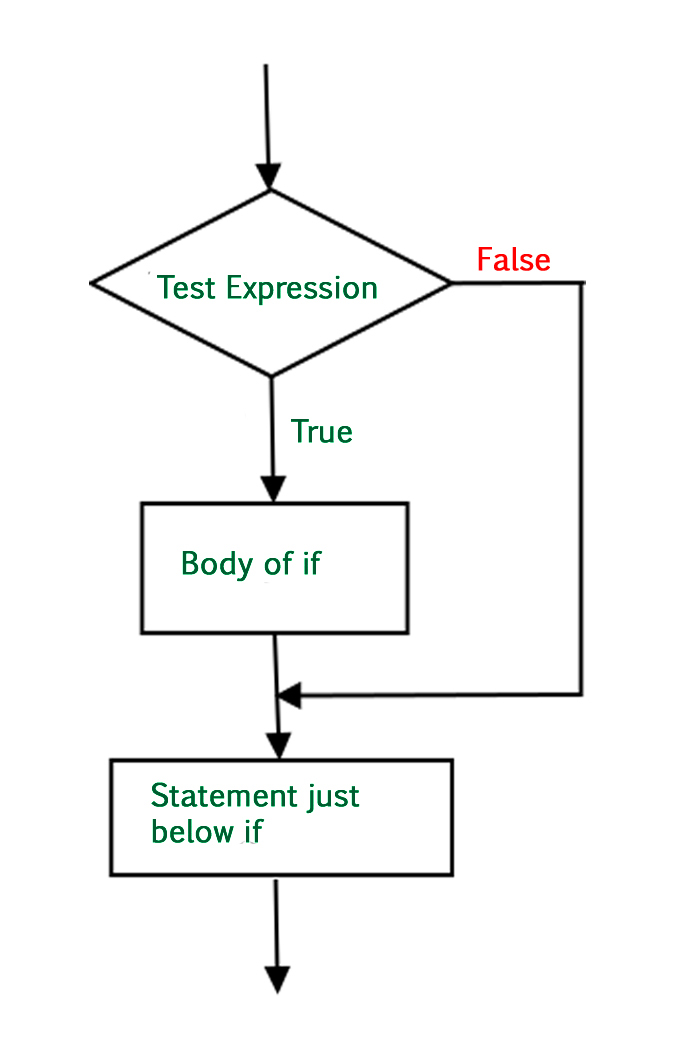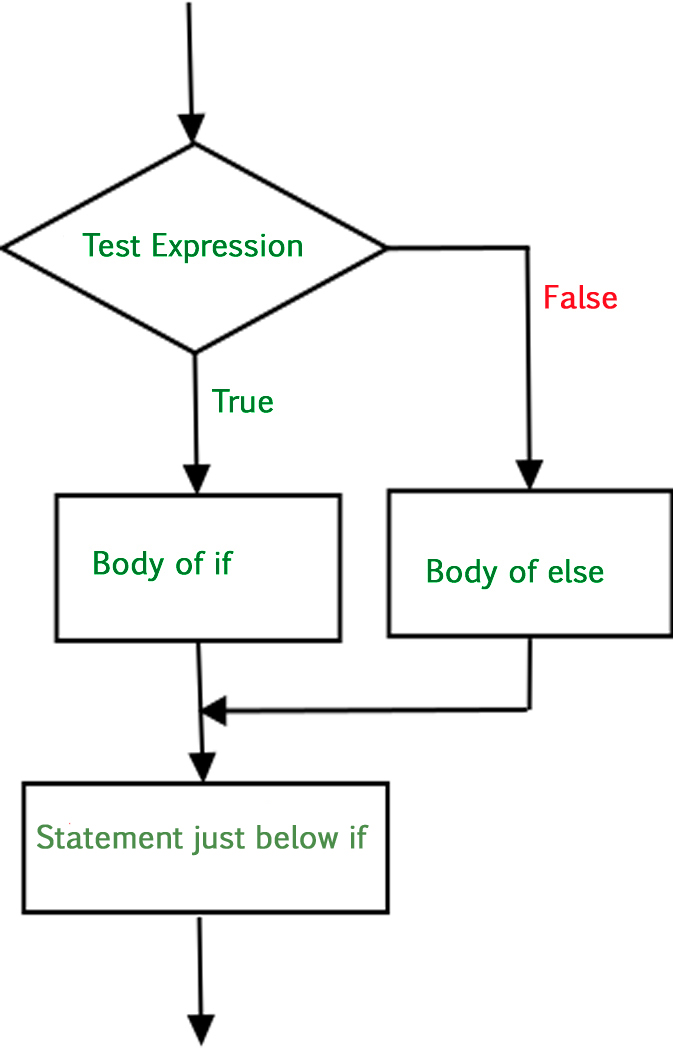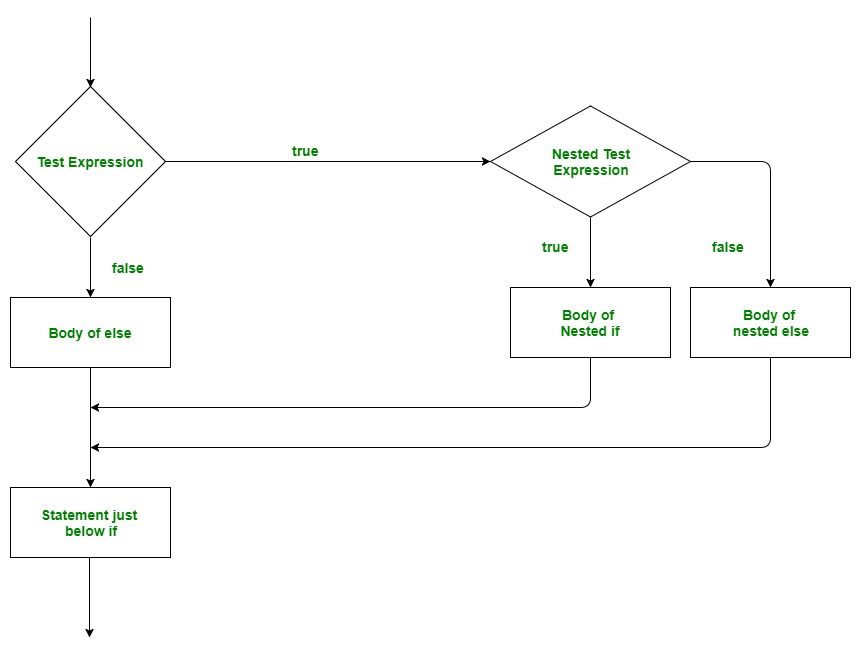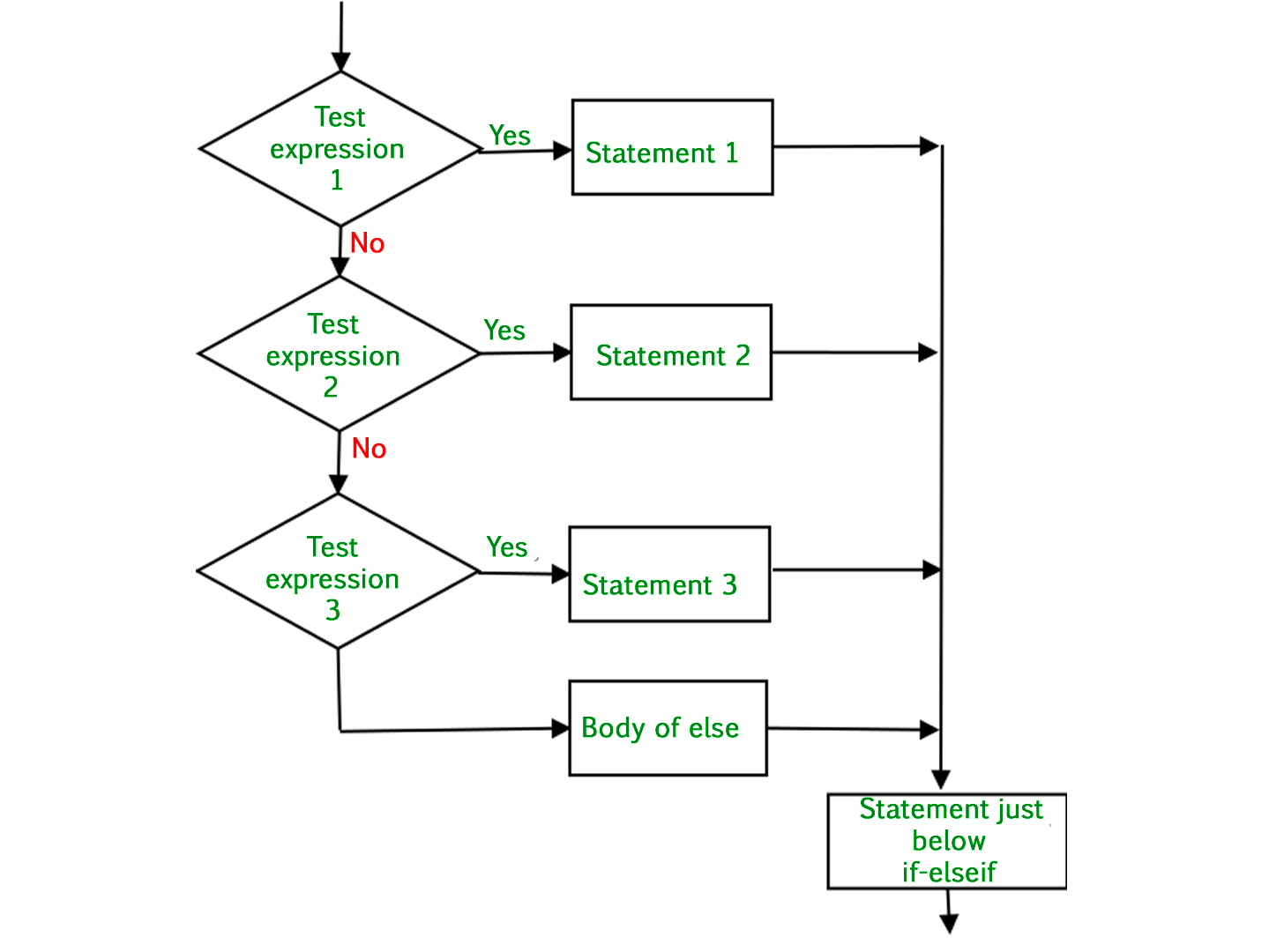# Control Flow in Python

• Difficulty Level : Easy
• Last Updated : 13 Jul, 2022

There comes situations in real life when we need to make some decisions and based on these decisions, we decide what should we do next. Similar situations arise in programming also where we need to make some decisions and based on these decisions we will execute the next block of code. Decision-making statements in programming languages decide the direction of the flow of program execution.

In Python, if-else elif statement is used for decision making.

## if statement

if statement is the most simple decision-making statement. It is used to decide whether a certain statement or block of statements will be executed or not i.e if a certain condition is true then a block of statement is executed otherwise not.

Syntax

```if condition:
# Statements to execute if
# condition is true```

Here, the condition after evaluation will be either true or false. if the statement accepts boolean values – if the value is true then it will execute the block of statements below it otherwise not. We can use condition with bracket ‘(‘ ‘)’ also.

As we know, python uses indentation to identify a block. So the block under an if statement will be identified as shown in the below example:

```if condition:
statement1
statement2

# Here if the condition is true, if block
# will consider only statement1 to be inside
# its block.```

## Flowchart of Python if statement## Python3

 `# python program to illustrate If statement`` ` `i ``=` `10`` ` `if` `(i > ``15``):``    ``print``(``"10 is less than 15"``)``print``(``"I am Not in if"``)`

Output:

`I am Not in if`

As the condition present in the if statement is false. So, the block below the if statement is executed.

## if-else

The if statement alone tells us that if a condition is true it will execute a block of statements and if the condition is false it won’t. But what if we want to do something else if the condition is false. Here comes the else statement. We can use the else statement with if statement to execute a block of code when the condition is false.

Syntax

```if (condition):
# Executes this block if
# condition is true
else:
# Executes this block if
# condition is false```

## FlowChart of Python if-else statement## Python3

 `# python program to illustrate If else statement``#!/usr/bin/python`` ` `i ``=` `20``if` `(i < ``15``):``    ``print``(``"i is smaller than 15"``)``    ``print``(``"i'm in if Block"``)``else``:``    ``print``(``"i is greater than 15"``)``    ``print``(``"i'm in else Block"``)``print``(``"i'm not in if and not in else Block"``)`

Output:

```i is greater than 15
i'm in else Block
i'm not in if and not in else Block```

The block of code following the else statement is executed as the condition present in the if statement is false after calling the statement which is not in block(without spaces).

## Python3

 `# Explicit function``def` `digitSum(n):``    ``dsum ``=` `0``    ``for` `ele ``in` `str``(n):``        ``dsum ``+``=` `int``(ele)``    ``return` `dsum`` ` ` ` `# Initializing list``List` `=` `[``367``, ``111``, ``562``, ``945``, ``6726``, ``873``]`` ` `# Using the function on odd elements of the list``newList ``=` `[digitSum(i) ``for` `i ``in` `List` `if` `i & ``1``]`` ` `# Displaying new list``print``(newList)`

Output

`[16, 3, 18, 18]`

## nested-if

A nested if is an if statement that is the target of another if statement. Nested if statements mean an if statement inside another if statement. Yes, Python allows us to nest if statements within if statements. i.e, we can place an if statement inside another if statement.

Syntax

```if (condition1):
# Executes when condition1 is true
if (condition2):
# Executes when condition2 is true
# if Block is end here
# if Block is end here```

## Flowchart of Python Nested if Statement## Python3

 `# python program to illustrate nested If statement``#!/usr/bin/python``i ``=` `10``if` `(i ``=``=` `10``):``   ` `    ``#  First if statement``    ``if` `(i < ``15``):``        ``print``(``"i is smaller than 15"``)``         ` `    ``# Nested - if statement``    ``# Will only be executed if statement above``    ``# it is true``    ``if` `(i < ``12``):``        ``print``(``"i is smaller than 12 too"``)``    ``else``:``        ``print``(``"i is greater than 15"``)`

Output:

```i is smaller than 15
i is smaller than 12 too```

Here, a user can decide among multiple options. The if statements are executed from the top down. As soon as one of the conditions controlling the if is true, the statement associated with that if is executed, and the rest of the ladder is bypassed. If none of the conditions is true, then the final else statement will be executed.

Syntax

```if (condition):
statement
elif (condition):
statement
.
.
else:
statement```

## FlowChart of Python if else elif statements## Python3

 `# Python program to illustrate if-elif-else ladder``#!/usr/bin/python`` ` `i ``=` `20``if` `(i ``=``=` `10``):``    ``print``(``"i is 10"``)``elif` `(i ``=``=` `15``):``    ``print``(``"i is 15"``)``elif` `(i ``=``=` `20``):``    ``print``(``"i is 20"``)``else``:``    ``print``(``"i is not present"``)`

Output:

`i is 20`

## Short Hand if statement

Whenever there is only a single statement to be executed inside the if block then shorthand if can be used. The statement can be put on the same line as the if statement.

Syntax:

`if condition: statement`

## Python3

 `# Python program to illustrate short hand if``i ``=` `10``if` `i < ``15``:``    ``print``(``"i is less than 15"``)`

Output:

`i is less than 15`

## Short Hand if-else statement

This can be used to write the if-else statements in a single line where there is only one statement to be executed in both if and else block.

Syntax:

`statement_when_True if condition else statement_when_False`

## Python3

 `# Python program to illustrate short hand if-else``i ``=` `10``print``(``True``) ``if` `i < ``15` `else` `print``(``False``)`

Output:

`True`

My Personal Notes arrow_drop_up